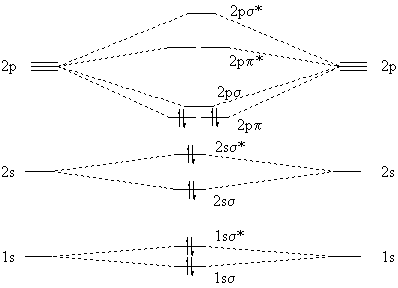# Solutions 22

$$\newcommand{\vecs}{\overset { \rightharpoonup} {\mathbf{#1}} }$$ $$\newcommand{\vecd}{\overset{-\!-\!\rightharpoonup}{\vphantom{a}\smash {#1}}}$$$$\newcommand{\id}{\mathrm{id}}$$ $$\newcommand{\Span}{\mathrm{span}}$$ $$\newcommand{\kernel}{\mathrm{null}\,}$$ $$\newcommand{\range}{\mathrm{range}\,}$$ $$\newcommand{\RealPart}{\mathrm{Re}}$$ $$\newcommand{\ImaginaryPart}{\mathrm{Im}}$$ $$\newcommand{\Argument}{\mathrm{Arg}}$$ $$\newcommand{\norm}{\| #1 \|}$$ $$\newcommand{\inner}{\langle #1, #2 \rangle}$$ $$\newcommand{\Span}{\mathrm{span}}$$ $$\newcommand{\id}{\mathrm{id}}$$ $$\newcommand{\Span}{\mathrm{span}}$$ $$\newcommand{\kernel}{\mathrm{null}\,}$$ $$\newcommand{\range}{\mathrm{range}\,}$$ $$\newcommand{\RealPart}{\mathrm{Re}}$$ $$\newcommand{\ImaginaryPart}{\mathrm{Im}}$$ $$\newcommand{\Argument}{\mathrm{Arg}}$$ $$\newcommand{\norm}{\| #1 \|}$$ $$\newcommand{\inner}{\langle #1, #2 \rangle}$$ $$\newcommand{\Span}{\mathrm{span}}$$

## Q22.1

We can calculate the transmittance:

$T = 1.00 - 0.9 = 0.10$

Also, the absorbance is

$A = -\log \left( \dfrac{T}{T_o} \right) = -\log (0.10) = 1.0$

Next step, use Beer-Lambert law to determine the molar absorptivity,

$\epsilon = \dfrac{A}{bc}$

$\epsilon = \dfrac{1}{(8.0\,cm)(5.0 \times 10^{-3}\, M)} = 25\, L \, mol^{-1} \, cm^{-1}$

## Q22.2

1. Use Heisenberg's uncertainty principle and the relationship between energy and frequency to find the uncertainty of frequency for a spectroscopic line.

$$\Delta E \Delta t \geq \dfrac{h}{4 \pi} \]$$ \Delta E = h \Delta \nu \]

$$h \Delta \nu \Delta t \geq \dfrac{h}{4 \pi} \]$$ \Delta \nu \geq \dfrac{1}{4 \pi \Delta t} \]

The maximum value for $$Δt$$ is the lifetime of the excited state.

$$\Delta \nu \geq \dfrac{1}{(4 \pi) \left(0.50 \, \cancel{ns} \times \dfrac{s}{10^9\ \cancel{ns}}\right)} \]$$ \Delta \nu \geq 1.6 \times 10^8\ s^{-1} \]

2. Use the uncertainty of frequency and the relationship between frequency and wavelength to find the uncertainty of the wavelength.

$$\lambda = \dfrac{c}{\nu} \]$$ | \Delta \lambda | = \dfrac{c}{\nu^2} | \Delta \nu | \]

This comes from propagation of error and then substituting the original equation back into it to get

$$| \Delta \lambda | = \dfrac{\lambda ^2 | \Delta \nu | }{c} \]$$ | \Delta \lambda | = \dfrac{ \left(430 \ \cancel{nm} \dfrac{m}{10^9\ \cancel{nm}} \right)^2 (1.6 \times 10^8\ s^{-1})}{2.998 \times 10^8\ m/s} \]

 | \Delta \lambda | = 9.87 \times 10^{-14}\ m \]

## Q22.3

1. Relaxation back to the $$S_o$$ ground state via fluorescence (i.e., emission without a spin flip)
2. Relaxation back to the $$S_o$$ ground state via internal conversion (i.e., no emission of a photon)
3. Intersystem crossing to the triplet manifold (e.g. a spin flip) resulting in the $$T_1$$ excited state.

## Q22.4

This question involves comparing the electron configuration of the excited $$C_2^*$$ molecule.

$(σ_{1s})^2(σ_{1s}^*)^2(σ_{2s})^2(σ_{2s}^*)^1(π_{2p_x})^2 (π_{2p_y})^2(σ_{2p})^1$

to the ground state $$C_2$$ molecule electron configuration

$(σ_{1s})^2(σ_{1s}^*)^2(σ_{2s})^2(σ_{2s}^*)^2(π_{2p_x})^2 (π_{2p_y})^2$

with the molecular orbital diagram shown below.Figure: Predicted molecular diagram and electron configuration for the ground state of $$C_2$$. Note that a slightly different nomenclature is used for the molecular orbitals. from Dan Berger (Bluffton College)

1. The bond order is given by $\text{Bond Order} = \dfrac{1}{2} \left[ (\text{number of bonding electron}) - (\text{number of anti-bonding electrons}) \right]$ So for $$C_2^*$$ with the electron configuration given this is $\text{Bond Order} = \dfrac{1}{2} \left[ 9 - 3 \right] = 3$ This is a triple bond.
2. The bond order for $$C_2$$ is then $\text{Bond Order} = \dfrac{1}{2} \left[ 8 - 4 \right] = 2$ This is a double bond.
3. Since the bonding is stronger (higher bond order) in the excited state, the bond is expected to be shorter.
4. Since the bonding is stronger (higher bond order) in the excited state, the bond energy is expected to be bigger.
5. Possible Paramagnetic or Diamagnetic since two unpaired electrons exist in the $$σ_{2s}^*$$ and $$σ_{2p}$$ orbitals. See answer to number 7.
6. Diamagnetic since each electron is paired.
7. You need to know if the singly occupied orbitals ( $$σ_{2s}^*$$ and $$σ_{2p}$$) have electrons that are either parallel to be a triplet state (e.g, Hund rules) or antiparallel to be a singlet state. That is, could they be either both up (or down) or one up/one down.

## Q22.5

The experimental fact is that $$C_2$$ has two electrons that are unpaired (paramagnetic). We explain this by noting that the $$2p_{\pi}$$ and $$2p_{\sigma}$$ molecular orbitals (termed $$π_{2p_x}/π_{2p_x}$$ and $$2\sigma_{p_z}$$, respectively, in the configurations above) are quite close in energy, so it takes more energy to pair the electrons than to put one in a slightly higher orbital. The resulting molecular-orbital model of $$C_2$$ isFigure: Experimental suggested molecular diagram and electron configuration for the ground state of $$C_2$$. Note that a slightly different nomenclature is used for the molecular orbitals. from Dan Berger (Bluffton College)

Notice that both molecular-orbital models show a net double bond between the carbon atoms (i.e., bond orders of 2).

## Q22.6

To be a good fluorophore, the competition between fluorescence, internal conversion and intersystem crossing has to favor fluorescence. That means the rate for fluorescence has to be faster than the other two and hence the timescales (1/rate) for the other two must be longer than the timescale associates with internal conversion and intersystem crossing.

Solutions 22 is shared under a not declared license and was authored, remixed, and/or curated by LibreTexts.